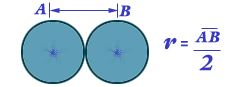##### Classification of Element and periodicity in properties

Ionic Radius Definition: The ionic radius is the measure of an atom's ion in a crystal lattice. Values for ionic radius are difficult to obtain and tend to depend on the method used to measure the size of the ion. A typical value for an ionic radius would be from 30 pm (0.3 Å) to 200 pm (2 Å).Ionic radius may be measured using x-ray crystallography or similar techniques.

The distance from the centre of the nucleus upto which it's outer most electron is extended.### For example:

C-C bond length in diamond is 1.54Ao.

Therefore, atomic radius of C = 1.54 Ao2

R = 0.77 Ao.

Atomic radius increases from top to bottom in a group.

### Reason:

Due to addition of an energy level.

Atomic radius decreases from left to right in a period.

### Reason:

Due to increase in nuclear charge.

ionic radius Half the distance between the ‘centres’ of two ions of the same element. Although no precise measurement can be made of the size of individual ions, in practice various techniques (e.g. X-ray diffraction) can be used to estimate ionic radii in particular crystal structures. Generally, it is found that:

(a) within the same group of the periodic table ionic radius increases with increasing atomic number;

(b) for elements of the same period (i.e. same horizontal row) that form positive ions, ionic radius decreases with increasing positive, for example, Na+ = 1.02, Mg2+ = 0.72, Al3+ = 0.53, Si4+ = 0.40;

(c) for the same reasons, if an element can exist in different valence states, the higher the positive charge the smaller the ion, for example, Mn2+ = 0.82, Mn3+ = 0.65;

(d) for elements of the same period forming negative ions, the ionic radius increases with increasing negative charge.